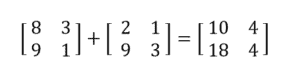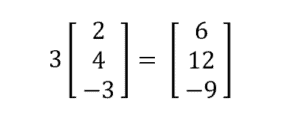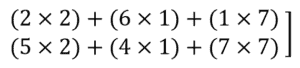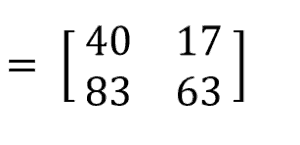Matrices

Organizing Data with a Matrix

In the first video, we are going to organize data with a matrix.

Let’s first define matrix:
Matrix: A rectangular array of data such as numbers and symbols.

For example:Let’s call the matrix A. Therefore:
A1,1 = 85 and
A3,2 = 86

Video-Lesson Transcript

Let’s look at organizing data with matrices.

First, let’s go over what matrix is.

Matrix is an array of data.

Look at this table where we have three different students, two different tests,

The matrix will just get the data and arrange it like this:$\begin{bmatrix} 85 & 81 \\ 91 & 93 \\ 74 & 86 \end{bmatrix}$

Here, we have three rows and two columns.

Dimensions are given by the number of rows by the number of columns or$rows \times columns$

So in this case, we have$3 \times 2$

Let’s look at the data again.

Each information is called an element. We can identify the element based on

its dimension or row and column.

Let’s call this example matrix A$\begin{bmatrix} 85 & 81 \\ 91 & 93 \\ 74 & 86 \end{bmatrix}$

Let’s label the rows and columns.$a_{1, 1} = 85$$a_{2, 1} = 91$$a_{3, 1} = 74$$a_{1, 2} = 81$$a_{2, 2} = 93$$a_{3, 2} = 86$

Adding and Subtracting Matrices

In the second video, we are going to add and subtract data with a matrix.

To do so, we can simply either add or subtract the values in the corresponding locations in the given matrices. Note that only matrices of the same size can be added or subtracted.

For example:And here is another oneVideo-Lesson Transcript

Let’s go over adding and subtracting matrices.

For example:$\begin{bmatrix} 3\\ 4 \end{bmatrix} - \begin{bmatrix} 6\\ 2 \end{bmatrix}$

We’re just going to subtract elements that are on the corresponding positions.$\begin{bmatrix} -3\\ 2 \end{bmatrix}$

Now, let’s have this$3 \times 2$ matrix. Three rows and two columns. Plus this other$3 \times 2$.$\begin{bmatrix} 8 & 3\\ 2 & 4\\ 9 & 1 \end{bmatrix} + \begin{bmatrix} 2 & 1\\ 3 & 6\\ 9 & 3 \end{bmatrix}$

So, our answer will be three rows and two columns.

Again, we’re just going to add corresponding elements.$\begin{bmatrix} 10 & 4\\ 5 & 10\\ 18 & 4 \end{bmatrix}$

Let’s look at a different example.

We’re given this problem:$\begin{bmatrix} 9 & 7\\ 3 & 2\\ 6 & 6 \end{bmatrix} + \begin{bmatrix} 2 & 8\\ 3 & 5 \end{bmatrix}$

What elements correspond to each other?

Which ones are we going to add together?

It’s difficult because one has a third row and the other doesn’t.

In this case, there is no match up.

So, there is no solution.

For addition and subtraction, the size or the dimension of the matrices have to be the same.

Multiplying a Matrix by a Scalar Number

In the third video, we are going to multiply a matrix by a scalar.

We can simply multiply the scalar number by each value inside the matrix.

For example:Video-Lesson Transcript

Let’s go over multiplying a matrix by a scalar.

Here we have a matrix to be multiplied by$3$.$3 \times \begin{bmatrix} 2\\ 4\\ -3 \end{bmatrix}$

If we multiply this matrix by a scalar which is$3$, our answer is going to be also$3 \times 1$.

So, let’s just multiply each element by$3$.$\begin{bmatrix} 6\\ 12\\ -9 \end{bmatrix}$

Let’s look at another example.$2 \times \begin{bmatrix} 5 & 4 & -2\\ -7 & 3 & 6 \end{bmatrix}$

Here, we have a scalar quantity$2$ and a two-row by three-column matrix.

When we multiply them, we’ll also end up with a two-row by a three-column matrix.

And just multiply each element by$2$.$\begin{bmatrix} 10 & 8 & -4\\ -14 & 6 & 12 \end{bmatrix}$

In multiplying a matrix by a scalar, we just have to multiply each element by that scalar quantity.

Multiplying a Matrix by a Matrix

In the fourth video, we are going to multiply a matrix with a matrix.

If the matrices have different sizes, multiply the corresponding numbers but of different orientations.

For example:Since the first matrix is 2×3 and the second matrix is 3×2, your answer is a 2×2 matrix. To get the value of$A_1,1$, multiply each number of the first row in the first matrix by the corresponding number in the first column of the second matrix, and add the products (Ex:2×5+6×4+1×6). To get the value of$A_1,2$, multiply each number of the first row in the first matrix by the corresponding number in the second column of the second matrix, and add the products. To get the value of$A_2,1$, multiply each number of the second row in the first matrix by the corresponding number in the first column of the second matrix, and add the products.  To get the value of$A_2,2$, multiply each number of the second row in the first matrix by the corresponding number in the second column of the second matrix, and add the products.Video-Lesson Transcript

Let’s go over multiplying a matrix by a matrix.

First thing I want to point out is that not all matrices can be multiplied together.

Let’s look at this example:$\begin{bmatrix} 2 & 6 & 1\\ 5 & 4 & 7 \end{bmatrix} \times \begin{bmatrix} 5 & 2\\ 4 & 1\\ 6 & 7 \end{bmatrix}$

Here, one matrix has two rows by three columns and the other one has three rows by two columns.

These two can be multiplied together.

You have check if the number of columns in the first matrix is the same as the number of rows in the second matrix.

So, yes, these can be multiplied together.

The result is what we’re left with. So, our answer will be a two by two matrix.

Multiplying matrix is a little bit complicated.

But we can do this!

First, let’s draw four spots or lines inside the matrix. Then label them as$1, 1$,$1, 2$,$2, 1$, and$2, 2$.

These labels represent the row and column.

To get what goes in each spot, we’re going to multiply the first row of the first matrix by the first column of the second matrix.

How do we do that?

We’re going to multiply the corresponding elements together.

In$1, 1$, we have$(2 \times 5) + (6 \times 4) + (1 \times 6)$

Now, we’re going to multiply the first row of the first matrix by the second column of the second matrix. To solve for$1, 2$ spot:$(2 \times 2) + (6 \times 1) + (1 \times 7)$

Now, we’re on the second row by first column. We’re going to multiply the second row of the first matrix by the first column of the second matrix.$(5 \times 4) + (4 \times 4) + (7 \times 6)$

In the second row by second column element, let’s multiply the second row of the first matrix by the second column of the second matrix.$(5 \times 2) + (4 \times 1) + (7 \times 7)$

Let’s use a calculator and our answer is$\begin{bmatrix} 40 & 17\\ 83 & 63 \end{bmatrix}$

A two by three matrix multiplied by three by two matrix gives us a two by two matrix.

Let’s look at this example,$\begin{bmatrix} 2\\ 4 \end{bmatrix} \times \begin{bmatrix} 3 & 6\\ 2 & 4 \end{bmatrix}$

Here, we have a two by one matrix multiplied by a two by two matrix.

Now, let’s look if the number of columns in the first matrix will match the number of rows in the second matrix.

It doesn’t.

First, the matrix has one column and the second matrix has two rows which don’t match up.

So, this one has no solution. We can’t multiply them together.

Let’s look at this one:$\begin{bmatrix} 3\\ 6\\ 4 \end{bmatrix} \times \begin{bmatrix} 2 & 1 \end{bmatrix}$

First matrix has three rows by one column. The second matrix has one row by two columns.

The number of columns in the first matrix match the number of rows in the second matrix.

So, we can multiply these two. And we’re going to end up with three by two matrix.

To solve, draw six spots or lines which will represent each element’s dimension.

First, multiply the first row by the first column$3 \times 2$

Next, we’ll multiply the second row by the first column$6 \times 2$

The next spot is for the third row by the first column$4 \times 2$

Then, let’s do the first row by the second column$3 \times 1$

Next spot is the second row by the second column$6 \times 1$

And lastly, we have the third row by the second column$4 \times 1$

And we end up with$\begin{bmatrix} 6 & 3\\ 12 & 6\\ 8 & 4 \end{bmatrix}$

Multiplying a three by one matrix by one by two matrix resulted in a three by two matrix.